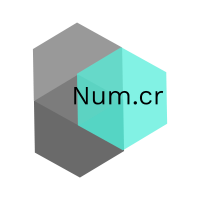# num.cr v0.4.5

Scientific computing in pure Crystal
• v0.4.5 - April 9, 2021
• v0.4.4 - November 25, 2020
• v0.4.3 - September 29, 2020
• 0.4.2 - August 5, 2020
• 0.4.1 - July 19, 2020Num.cr is the core shard needed for scientific computing with Crystal

It provides:

• An n-dimensional `Tensor` data structure
• Efficient `map`, `reduce` and `accumulate` routines
• GPU accelerated routines backed by `OpenCL`
• Linear algebra routines backed by `LAPACK` and `BLAS`

## Prerequisites

`Num.cr` aims to be a scientific computing library written in pure Crystal. All standard operations and data structures are written in Crystal. Certain routines, primarily linear algebra routines, are instead provided by a `BLAS` or `LAPACK` implementation.

Several implementations can be used, including `Cblas`, `Openblas`, and the `Accelerate` framework on Darwin systems. For GPU accelerated `BLAS` routines, the `ClBlast` library is required.

`Num.cr` also supports `Tensor`s stored on a `GPU`. This is currently limited to `OpenCL`, and a valid `OpenCL` installation and device(s) are required.

## Installation

Add this to your applications `shard.yml`

``````dependencies:
num:
github: crystal-data/num.cr
``````

Several third-party libraries are required to use certain features of `Num.cr`. They are:

• BLAS
• LAPACK
• OpenCL
• ClBlast

While not at all required, they provide additional functionality than is provided by the basic library.

## Just show me the code

The core data structure implemented by `Num.cr` is the `Tensor`, an N-dimensional data structure. A `Tensor` supports slicing, mutation, permutation, reduction, and accumulation. A `Tensor` can be a view of another `Tensor`, and can support either C-style or Fortran-style storage.

### Creation

There are many ways to initialize a `Tensor`. Most creation methods can allocate a `Tensor` backed by either `CPU` or `GPU` based storage.

``````[1, 2, 3].to_tensor
Tensor.from_array [1, 2, 3]
Tensor(UInt8).zeros([3, 3, 2])
Tensor.random(0.0...1.0, [2, 2, 2])

ClTensor(Float32).zeros([3, 2, 2])
ClTensor(Float64).full([3, 4, 5], 3.8)
``````

### Operations

A `Tensor` supports a wide variety of numerical operations. Many of these operations are provided by `Num.cr`, but any operation can be mapped across one or more `Tensor`s using sophisticated broadcasted mapping routines.

``````a = [1, 2, 3, 4].to_tensor
b = [[3, 4, 5, 6], [5, 6, 7, 8]].to_tensor

# Convert a Tensor to a GPU backed Tensor
acl = a.astype(Float64).gpu

puts Num.add(a, b)

# a is broadcast to b's shape
# [[ 4,  6,  8, 10],
#  [ 6,  8, 10, 12]]
``````

When operating on more than two `Tensor`s, it is recommended to use `map` rather than builtin functions to avoid the allocation of intermediate results. All `map` operations support broadcasting.

``````a = [1, 2, 3, 4].to_tensor
b = [[3, 4, 5, 6], [5, 6, 7, 8]].to_tensor
c = [3, 5, 7, 9].to_tensor

a.map(b, c) do |i, j, k|
i + 2 / j + k * 3.5
end

# [[12.1667, 20     , 27.9   , 35.8333],
#  [11.9   , 19.8333, 27.7857, 35.75  ]]
``````

### Mutation

`Tensor`s support flexible slicing and mutation operations. Many of these operations return views, not copies, so any changes made to the results might also be reflected in the parent.

``````a = Tensor.new([3, 2, 2]) { |i| i }

puts a.transpose

# [[[ 0,  4,  8],
#   [ 2,  6, 10]],
#
#  [[ 1,  5,  9],
#   [ 3,  7, 11]]]

puts a.reshape(6, 2)

# [[ 0,  1],
#  [ 2,  3],
#  [ 4,  5],
#  [ 6,  7],
#  [ 8,  9],
#  [10, 11]]

puts a[..., 1]

# [[ 2,  3],
#  [ 6,  7],
#  [10, 11]]

puts a[1..., {..., -1}]

# [[[ 6,  7],
#   [ 4,  5]],
#
#  [[10, 11],
#   [ 8,  9]]]

puts a[0, 1, 1].value

# 3
``````

### Linear Algebra

`Tensor`s provide easy access to power Linear Algebra routines backed by LAPACK and BLAS implementations, and ClBlast for GPU backed `Tensor`s.

``````a = [[1, 2], [3, 4]].to_tensor.map &.to_f32

puts a.inv

# [[-2  , 1   ],
#  [1.5 , -0.5]]

puts a.eigvals

# [-0.372281, 5.37228  ]

acl = a.opencl
bcl = a.opencl

puts acl.gemm(bcl).cpu

# [[7 , 10],
#  [15, 22]]

puts a.matmul(a)

# [[7 , 10],
#  [15, 22]]
``````

### Machine Learning

`Num::Grad` provides a pure-crystal approach to find derivatives of mathematical functions. Use a `Num::Grad::Variable` with a `Num::Grad::Context` to easily compute these derivatives.

``````ctx = Num::Grad::Context(Tensor(Float64)).new

x = ctx.variable([3.0])
y = ctx.variable([2.0])

# f(x) = x ** y
f = x ** y
puts f # => 

f.backprop

# df/dx = y * x = 6.0
puts x.grad # => [6.0]
``````

`Num::NN` contains an extension to `Num::Grad` that provides an easy-to-use interface to assist in creating neural networks. Designing and creating a network is simple using Crystal's block syntax.

``````ctx = Num::Grad::Context(Tensor(Float64)).new

x_train = [[0.0, 0.0], [1.0, 0.0], [0.0, 1.0], [1.0, 1.0]].to_tensor
y_train = [[0.0], [1.0], [1.0], [0.0]].to_tensor

x = ctx.variable(x_train)

net = Num::NN::Network.new(ctx) do
input 
# A basic network with a single hidden layer using
# a ReLU activation function
linear 3
relu
linear 1

# SGD Optimizer
sgd 0.7

# Sigmoid Cross Entropy to calculate loss
sigmoid_cross_entropy_loss
end

500.times do |epoch|
y_pred = net.forward(x)
loss = net.loss(y_pred, y_train)
puts "Epoch: #{epoch} - Loss #{loss}"
loss.backprop
net.optimizer.update
end

# Clip results to make a prediction
puts net.forward(x).value.map { |el| el > 0 ? 1 : 0}

# [,
#  ,
#  ,
#  ]
``````

Review the documentation for full implementation details, and if something is missing, open an issue to add it!

Owner
github statistic
• 92
• 6
• 7
• 4
• 2 months ago
• October 10, 2019
License

MIT License

Links
Synced at

Tue, 22 Jun 2021 03:12:31 GMT